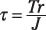##### Mechanics of Materials For Dummies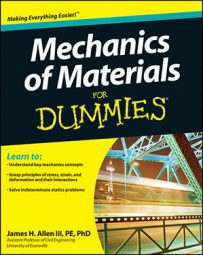When dealing with mechanics of materials, choosing the correct formula to calculate the stress at a given point can be difficult. Normal and shear stresses come in a wide variety of applications, each stress application with its own calculation formula. The most common stress types you deal with in basic mechanics of materials fall into several major categories:

• Axial tension: ANET is equal to the gross area of the cross section minus any holes that may exist.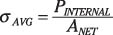• Thin-walled pressure vessels: Two stresses exist: an axial stress along the axis of the member and a hoop (or radial) stress, which occurs tangential to the radius of the cross section. These stresses are based on the gage pressure p inside the pressure vessel.

For cylindrical pressure vessels, use this pair of formulas: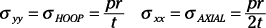For spherical pressure vessels, use the following formula: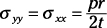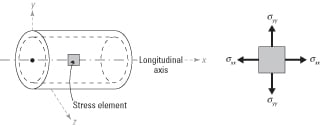• Bending stresses: For symmetric cross sections in the XY plane, use this formula: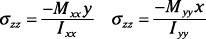(moment about the x-axis)(moment about the y-axis)

• Flexural shear stresses: Here's the formula for calculating flexural shear stress: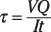• Torsional shear stress: Use this formula to find torsional shear stress: